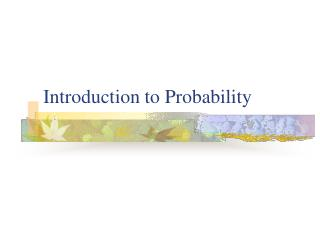# Introduction to Probability - PowerPoint PPT PresentationDownload PresentationIntroduction to Probability

Download Presentation## Introduction to Probability

- - - - - - - - - - - - - - - - - - - - - - - - - - - E N D - - - - - - - - - - - - - - - - - - - - - - - - - - -
##### Presentation Transcript

1. Introduction to Probability

2. Definitions The sample space is a set S composed of all the possible outcomes of an experiment. • If we flip a coin twice, the sample space might be taken as S = {hh, ht, th, tt} • The sample space for the outcomes of the 2000 US presidential election might be taken as S = {Bush, Gore, Nader, Buchanan}

3. Definitions (continued) An event is a subset of the sample space, or A  S is an “event”. • A = {hh} would be the event that heads occur twice when a coin is flipped twice. • A = {Gore, Bush} would be the event that a major party candidate wins the election.

4. Probability • For every event A  S, Pr(A)  0 • Σpi = 1 • All of the probabilities constitute the probability distribution. • For all A  S, Pr(A) + Pr(Ā) = 1

5. Statistical Independence H is statistically independent of G if: Pr(HG) = Pr(H) Recall that Pr(H and G) = Pr(G)Pr(HG) If H and G are independent, then we can replace Pr(HG) with Pr(H)

6. Statistical Independence Thus for independent events, Pr(H and G) = Pr(G)Pr(H) Example: the probability of having a boy, girl, and then boy in a family of three The sex of each child is independent of the sex of the others, thus we can calculate Pr(B and G and B) = Pr(B)Pr(G)Pr(B) Pr(B and G and B) = (1/2)(1/2)(1/2) = 1/8

7. Review of Probability Rules • Pr(G or H) = Pr(G) + Pr(H) - Pr(G and H); for mutually exclusive events, Pr(G and H) = 0 • Pr(G and H) = Pr(G)Pr(HG), also written as Pr(HG) = Pr(G and H)/Pr(G) • If G and H are independent then, Pr(HG) = Pr(H), thus Pr(G and H) = Pr(G)Pr(H)

8. Odds Another way to express probability is in terms of odds, d d = p/(1-p) p = probability of an outcome

9. Odds (continued) Example: What are the odds of getting a six on a dice throw? We know that p=1/6, so d = 1/6/(1-1/6) = (1/6)/(5/6) = 1/5. Gamblers often turn it around and say that the odds against getting a six on a dice roll are 5 to 1.

10. Bayes Theorem Sometimes we have prior information or beliefs about the outcomes we expect. Example: I am thinking of buying a used Saturn at Honest Ed's. I look up the record of Saturns in an auto magazine and find that, unfortunately, 30% of these cars have faulty transmissions. To get a better estimate of the particular car I want to purchase, I hire a mechanic who can make a guess on the basis of a quick drive around the block. I know that the mechanic is able to pronounce 90% of the faulty cars faulty and he is able to pronounce 80% of the good cars good.

11. Bayes Theorem (continued) What is the chance that the Saturn I'm thinking of buying has a faulty transmission: 1) Before I hire the mechanic? 2) If the mechanic pronounces it faulty? 3) If the mechanic pronounces it ok?

12. Bayes Theorem (continued) We can represent this information in a decision tree. Using Bayes Theorem: Pr(AMA) = Pr(A)Pr(MAA) Pr(A)Pr(MAA) + Pr(Ā)Pr(MA Ā) A = transmission is actually faulty MA= mechanic declares transmission faulty

13. Bayes Theorem (continued) Pr(A) = .3 Pr(MAA) = .9 Pr(Ā) = .7 Pr(MAĀ) = .2 Pr(AMA) = (.3)(.9) = .27/.41 = .659 (.3)(.9) + (.7)(.2)

14. Bayes Theorem (continued) Interpretation: The probability that the transmission is faulty if the mechanic declares it faulty is 0.659, which is a much better estimate than our guess based on the auto magazine (0.3).

15. Another Example What is the probability that the transmission is faulty if the mechanic claims it is good? Pr(AM Ā) = Pr(A)Pr(M ĀA) Pr(A)Pr(M ĀA) + Pr(Ā)Pr(M Ā Ā) Pr(AM Ā) = (.3)(.1) = .3/.59 = .051 (.3)(.1) + (.7)(.8)

16. Another Example (continued) Interpretation: The probability that the transmission is faulty if the mechanic declares it good is only .051, quite small.

17. Generalizing Bayes Theorem We can generalize Bayes Theorem to problems with more than 2 outcomes. Suppose there are 3 nickel sized coins in a box. Coin 1 Coin 2 Coin 3 2 headed 2 tailed fair

18. Generalizing Bayes Theorem You reach in and grab a coin at random and flip it without looking at the coin. It comes up heads. What is the probability that you have drawn the two headed coin (#1)? Pr(2Hhead) = Pr(2H)Pr(head2H) Pr(2H)Pr(head2H) + Pr(fair)Pr(headfair) + Pr(2T)Pr(head2T) Pr(2Hhead) = (1/3)(1) = (1/3)/(1/2) = 2/3 = .667 (1/3)(1) + (1/3)(1/2) + (1/3)(0)